Home > ACC7 > Chapter cc310 > Lesson cc310.1.5 > Problem10-69

10-69.
1. First decide if the triangles below are similar. Then, if they are similar, use the information in each diagram to solve for x. Homework Help ✎

1.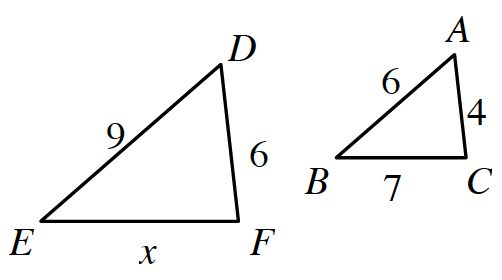2.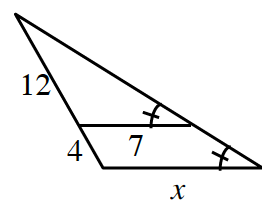Careful!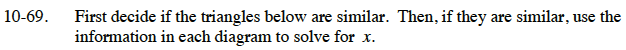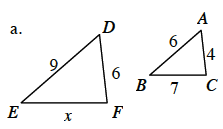If only two pair of corresponding sides have equal ratios, can you conclude that the triangles are similar?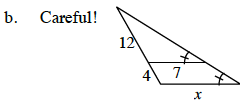The two triangles are similar (by AA~).

Draw two separate triangles, then write a proportion and solve for x.

$\frac{12}{7}=\frac{16}{x}$